# Explain The Working Principle of MOS Transistor

## MOS Transistor

The Complementary MOSFET (CMOS) technology is widely used today to form circuits in almost all applications.

Now a days all computers, CPUs and cell phones make use of CMOS due to several key advantages. Such as :

1. Its low power dissipation
2. Relatively high speed
3. High noise margins in both states
4. It can operate over a wide range of source and input voltages (provided the source voltage is fixed)

In this article, we will discuss, the type of transistor available is the Metal-Oxide-Semiconductor Field Effect Transistor (MOSFET).

These transistors are formed as a ‘sandwich’ consisting of a semiconductor layer (usually a slice, or wafer) made from a single crystal of silicon; a layer of silicon dioxide (the oxide) and a layer of metal.

### Structure of a MOSFET

As shown in the fig.1, MOS structure contains three layers:

1. The Metal Gate Electrode
2. The Insulating Oxide Layer (SiO2)
3.  P – type Semiconductor (Substrate)

This MOS structure forms a capacitor, with gate and substrate are as two plates and oxide layer as the dielectric material.

The thickness of dielectric material (SiO2) is usually between 10 nm and 50 nm.

Carrier concentration and distribution within the substrate can be manipulated by external voltage applied to gate and substrate terminal.

Now, to understand the structure of MOS, first consider the basic electric properties of P – Type semiconductor substrate.

Concentration of carrier in semiconductor material is always following the Mass Action Law. Mass Action Law is given by: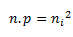Where,

• n is carrier concentration of electrons
• p is carrier concentration of holes
• ni is intrinsic carrier concentration of Silicon

Now assume that substrate is equally doped with acceptor (Boron) concentration NA. So, electron and hole concentration in p–type substrate is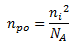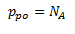Here, doping concentration NA is (1015 to 1016 cm-3 ) greater than intrinsic concentration ni .

Now, to understand the MOS structure, consider the energy level diagram of p–type silicon substrate.

As shown in the fig.2, the band gap between conduction band and valance band is 1.1eV.

Here, Fermi potential ΦF is the difference between intrinsic Fermi level (Ei) and Fermi level (EFp).

Where Fermi level Edepends on the doping concentration.

Fermi potential  Φis the difference between intrinsic Fermi level (Ei) and Fermi level (EFp). Mathematically,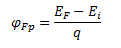The potential difference between conduction band and free space is called electron affinity and is denoted by qx .

So, energy required for an electron to move from Fermi level to free space is called work function (qϕs) and it is given by: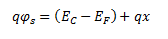The following fig.3 shows the energy band diagram of components that make up the MOS.

As shown in the above figure, insulating SiO2 layer has large energy band gap of 8 eV and work function is 0.95 eV. Metal gate has work function of 4.1eV. Here, the work functions are different so it will create voltage drop across the MOS system.

The figure given below shows the combined energy band diagram of MOS system.

As shown in this figure, the fermi potential level of metal gate and semiconductor (Si) are at same potential.

Fermi potential at surface is called surface potential ΦS and it is smaller than Fermi potential ΦF in magnitude.

### Working of a MOSFET

MOSFET consists of a MOS capacitor with two p-n junctions placed closed to the channel region and this region is controlled by gate voltage.

To make both the p-n junction reverse biased, substrate potential is kept lower than the other three terminals potential.

If the gate voltage will be increased beyond the threshold voltage (VGS >VTO), inversion layer will be established on the surface and n – type channel will be formed between the source and drain.

This n – type channel will carry the drain current according to the VDS value.

For different value of VDS, MOSFET can be operated in different regions as explained below.

### Linear Region

At VDS = 0, thermal equilibrium exists in the inverted channel region and drain current ID = 0.

Now if small drain voltage, VDS > 0 is applied, a drain current proportional to the VDS will start to flow from source to drain through the channel.

The channel gives a continuous path for the flow of current from source to drain.

This mode of operation is called linear region.

The cross sectional view of an n-channel MOSFET, operating in linear region, is shown in the figure given below.

### At the Edge of Saturation Region

Now if the VDS is increased, charges in the channel and the channel depth decrease at the end of drain.

For VDS = VDSSAT, the charges in the channel is reduces to zero, which is called pinch – off point.

The cross sectional view of n-channel MOSFET operating at the edge of saturation region is shown in the figure given below.

### Saturation Region

For VDS >VDSSAT, a depleted surface forms near to drain, and by increasing the drain voltage this depleted region extends to source.

This mode of operation is called Saturation region.

The electrons coming from the source to the channel end, enter in the drain – depletion region and are accelerated towards the drain in high electric field.